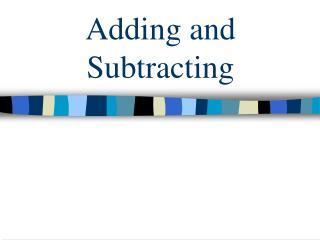DownloadDownload PresentationTélécharger la présentation- - - - - - - - - - - - - - - - - - - - - - - - - - - E N D - - - - - - - - - - - - - - - - - - - - - - - - - - -
##### Presentation Transcript

2. Adding and Subtracting… • The important thing to remember about adding or subtracting is that the exponents must be the same! • If the exponents are not the same then it is necessary to change one of the numbers so that both numbers have the same exponential value.

3. Adding… • The general format for adding is as follows… • (N x 10x) + (M x 10x) = (N + M) x 10x • The first step, if necessary, is to change one of the numbers so that both numbers have the same exponential value.

4. Adding… • Secondly, add the N and M numbers together and express as an answer. • The final step is to multiply the result by the 10x. • (It may be necessary to put the resulting answer into proper scientific notation.)

5. Adding With the Same Exponent • (3.45 x 103) + (6.11 x 103) • 3.45 + 6.11 = 9.56 • 9.56 x 103

6. Adding With Different Exponents • (4.12 x 106) + (3.94 x 104) • (412 x 104) + (3.94 x 104) • 412 + 3.94 = 415.94 • 415.94 x 104 • Express in proper form: 4.15 x 106

7. Now it’s your turn. • Use the link below to practice adding numbers in scientific notation. • Adding Numbers in Scientific Notation

8. Subtracting… • The general form for subtracting is as follows… • (N x 10x) – (M x 10x) = (N – M) x 10x • The first step, if necessary is to change one of the numbers so that both numbers have the same exponential value. (Just like adding).

9. Subtracting… • Secondly, subtract the M number from the N number and express as an answer. • The final step is to multiply the result by the 10x. • (It may be necessary to put the resulting answer into proper scientific notation.)

10. Subtracting With the Same Exponent • (8.96 x 107) – (3.41 x 107) • 8.96 – 3.41 = 5.55 • 5.55 x 107

11. Subtracting With Different Exponents • (4.23 x 103) – (9.56 x 102) • (42.3 x 102) – (9.56 x 102) • 42.3 – 9.56 = 32.74 • 32.74 x 102 • Express in proper form: 3.27 x 103

12. Now it’s your turn. • Use the link below to practice subtracting numbers in scientific notation. • Subtracting Numbers in Scientific Notation

13. Quiz Time!!! • Below is a set of links for a quiz on adding and subtracting numbers in scientific notation, and there is a link to get the answers to the quiz. • Adding and Subtracting Quiz • Answers to Quiz